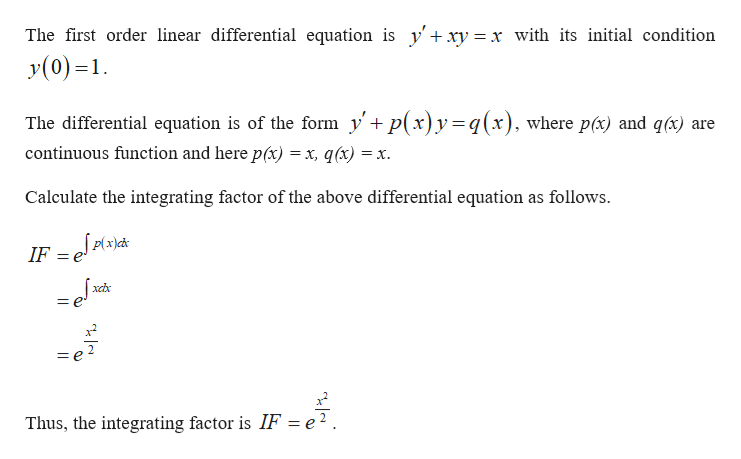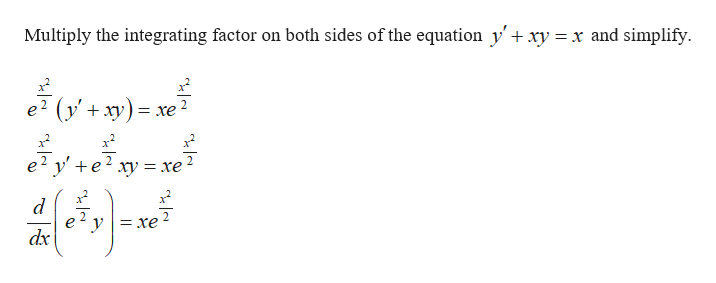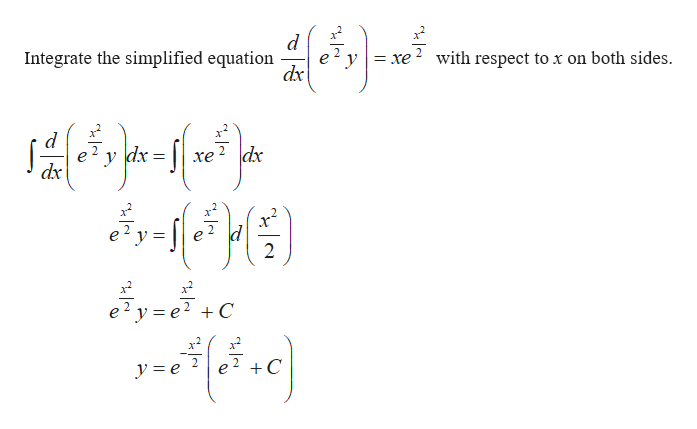# Use the method of integrating factors to solve the initial value problem.y'+xy=x, y(0)=1

Question
1 views

Use the method of integrating factors to solve the initial value problem.

• y'+xy=x, y(0)=1
check_circle

Step 1help_outlineImage TranscriptioncloseThe first order linear differential equation is y'+xy = x with its initial condition y(0)=1 The differential equation is of the form yp(x)y=q(x), where pc) and q(x) + are continuous function and here p(x) = x, q(x) = X. Calculate the integrating factor of the above differential equation as follows |p(x)cd* IF = J = e Thus, the integrating factor is IF = e2. fullscreen
Step 2help_outlineImage TranscriptioncloseMultiply the integrating factor on both sides of the equation y'+ xy =x and simplify. e2 yxy) =xe2 x e2 y'e2 xy = xe2 e dx fullscreen
Step 3help_outlineImage Transcriptionclose= xe2 with respect to x on both sides Integrate the simplified equation e у dx d dx хе dx 2 е? у 3е? +С 2 C 2 y e fullscreen

### Want to see the full answer?

See Solution

#### Want to see this answer and more?

Solutions are written by subject experts who are available 24/7. Questions are typically answered within 1 hour.*

See Solution
*Response times may vary by subject and question.
Tagged in

### Math